6.5.1 Step 2: Delete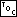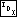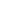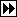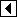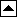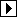At this point, p is the node to be deleted and the stack contains all of the nodes on the simple path from the tree's root down to p. The immediate task is to delete p. We break deletion down into the familiar three cases (see Deleting from a BST), but before we dive into the code, let's think about the situation.

In red-black insertion, we were able to limit the kinds of violation that could occur to rule 1 or rule 2, at our option, by choosing the new node's color. No such luxury is available in deletion, because colors have already been assigned to all of the nodes. In fact, a naive approach to deletion can lead to multiple violations in widely separated parts of a tree. Consider the effects of deletion of node 3 from the following red-black tree tree, supposing that it is a subtree of some larger tree: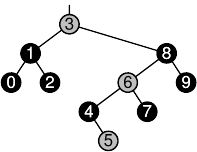If we performed this deletion in a literal-minded fashion, we would end up with the tree below, with the following violations: rule 1, between node 6 and its child; rule 2, at node 6; rule 2, at node 4, because the black-height of the subtree as a whole has increased (ignoring the rule 2 violation at node 6); and rule 1, at node 4, only if the subtree's parent is red. The result is difficult to rebalance in general because we have two problem areas to deal with, one at node 4, one at node 6.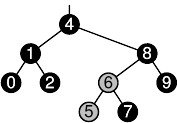Fortunately, we can make things easier for ourselves. We can eliminate the problem area at node 4 simply by recoloring it red, the same color as the node it replaced, as shown below. Then all we have to deal with are the violations at node 6: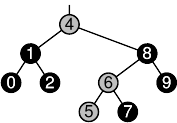This idea holds in general. So, when we replace the deleted node p by a different node q, we set q's color to p's. Besides that, as an implementation detail, we need to keep track of the color of the node that was moved, i.e., node q's former color. We do this here by saving it temporarily in p. In other words, when we replace one node by another during deletion, we swap their colors.

Now we know enough to begin the implementation. While reading this code, keep in mind that after deletion, regardless of the case selected, the stack contains a list of the nodes where rebalancing may be required, and da[k - 1] indicates the side of pa[k - 1] from which a node of color p->rb_color was deleted. Here's an outline of the meat of the code:

```221. <Step 2: Delete item from RB tree 221> =
if (p->rb_link == NULL)
{ <Case 1 in RB deletion 222> }
else   {
enum rb_color t;
struct rb_node *r = p->rb_link;

if (r->rb_link == NULL)
{         <Case 2 in RB deletion 223>       }
else       {         <Case 3 in RB deletion 224>       }
}
```

This code is included in 220.

##### Case 1: p has no right child

In case 1, p has no right child, so we replace it by its left subtree. As a very special case, there is no need to do any swapping of colors (see Exercise 1 for details).

```222. <Case 1 in RB deletion 222> =
pa[k - 1]->rb_link[da[k - 1]] = p->rb_link;
```

This code is included in 221.

##### Case 2: p's right child has no left child

In this case, p has a right child r, which in turn has no left child. We replace p by r, swap the colors of nodes p and r, and add r to the stack because we may need to rebalance there. Here's a pre- and post-deletion diagram that shows one possible set of colors out of the possibilities. Node p is shown detached after deletion to make it clear that the colors are swapped: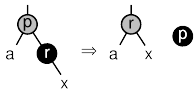```223. <Case 2 in RB deletion 223> =
t = r->rb_color;
r->rb_color = p->rb_color;
p->rb_color = t;
pa[k - 1]->rb_link[da[k - 1]] = r;
da[k] = 1;
pa[k++] = r;
```

This code is included in 221.

##### Case 3: p's right child has a left child

In this case, p's right child has a left child. The code here is basically the same as for AVL deletion. We replace p by its inorder successor s and swap their node colors. Because they may require rebalancing, we also add all of the nodes we visit to the stack. Here's a diagram to clear up matters, again with arbitrary colors: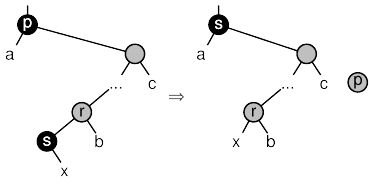```224. <Case 3 in RB deletion 224> =
struct rb_node *s;
int j = k++;

for (;;)   {
da[k] = 0;
pa[k++] = r;
if (s->rb_link == NULL)
break;

r = s;
}

da[j] = 1;
pa[j] = s;
pa[j - 1]->rb_link[da[j - 1]] = s;